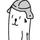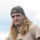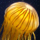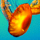## General Question# How to calculate median of a list of numbers in Scheme (Lisp)?

Asked by zarnold (695) October 11th, 2007
Observing members: 0Composing members: 0I don’t know Scheme at all.

Logically I’d say;
Sort the list.
If the number of items is odd, the median will be in the middle position. (middle position for an odd number would be ((NumInList – 1 ) / 2 ) + 1
for an even number the median is the two middle positions averaged together.

Something like :

sort list.
if (numInList odd) >> median =( ( numInList -1 ) / 2 ) + 1
else ( numList even) >> median = ( list[ numlist/2 -1 ] + list[numlist/2] ) / 2

where list[n] lets you access the nth position in the list and numList is the number in the list. (note the positions for the even number list may vary depending on whether your list starts at position 0 or 1)

Don’t know how to translate this into Scheme, but I hope this helped!

Perchik (4982)“Great Answer” (1) Flag as…thanks alot Perchik…I don’t think translating it should be too hard. I’ll just play around with Drscheme until it works :)

zarnold (695)“Great Answer” (0) Flag as…Heh I just noticed that I used “numInList” and “numList” those both should be the same thing and it should be the number of items in the list.

Perchik (4982)“Great Answer” (0) Flag as…Forgive the poor markup. This is a Lisp solution (I think, it’s been a while since I’ve dealt with the median function).

(defun median (list)
(let ((sorted (sort list #’<))
(i (round (/ (length list) 2))))
(nth i sorted)))

I couldn’t figure out how to sort a list in DrScheme but I’m sure it’ll be an easy port.

FusionGyro (82)“Great Answer” (3) Flag as…how to calculate the length of a list in scheme ?

sash (1)“Great Answer” (0) Flag as…how to use cons,car,cdr in scheme give it with example

sash (1)“Great Answer” (0) Flag as…or